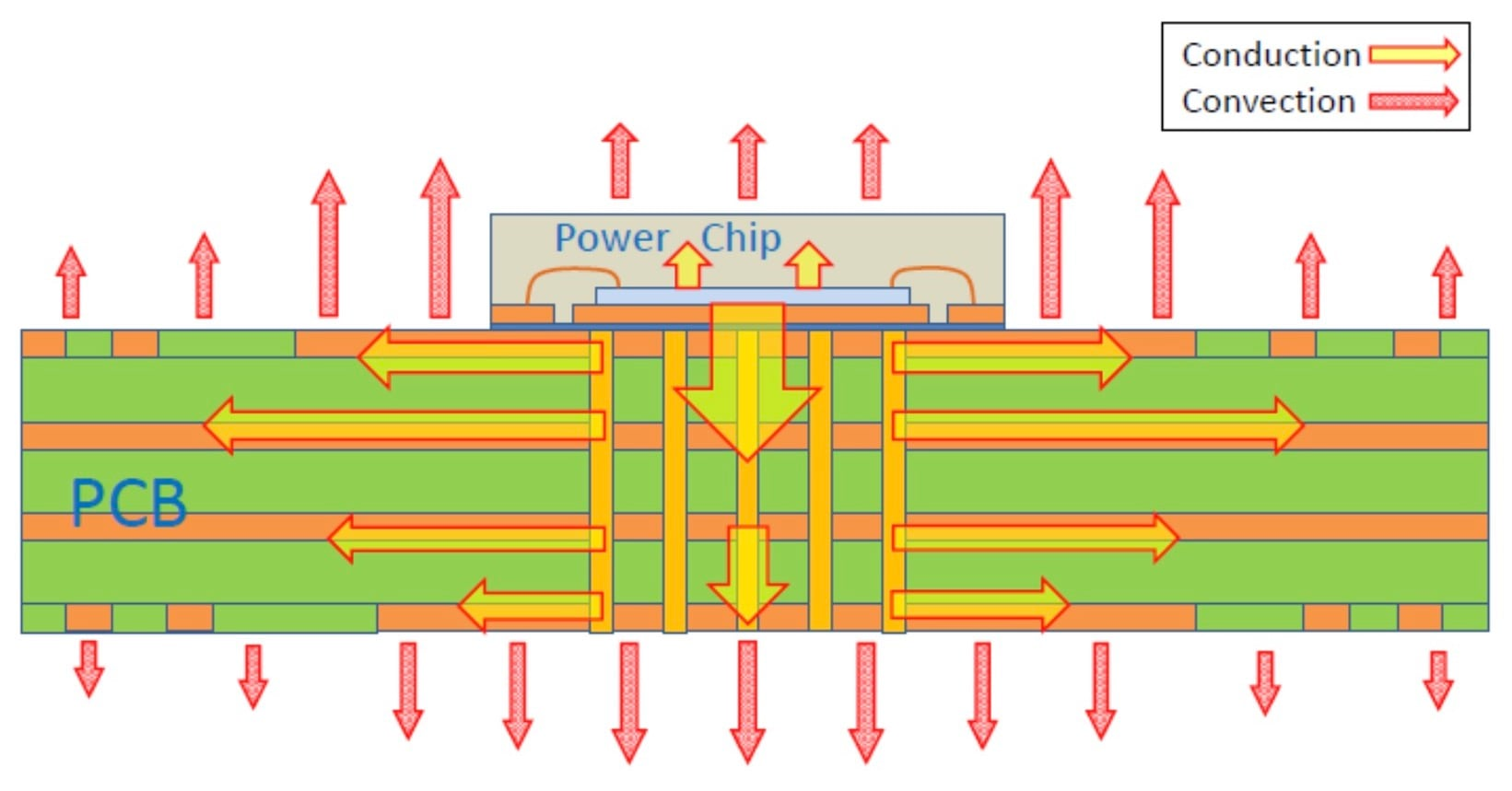Technical Article

# How Junction-to-Ambient Thermal Resistance of an IC Package Affects Thermal Performance

December 27, 2020 by Dr. Steve Arar

## Assessing the thermal performance of an IC package becomes easier if you understand this common, but often misapplied, parameter known as theta JA.

Thermal performance of an IC (integrated circuit) package can be specified by several different parameters such as θJA, θJB, θJC, ΨJT, and ΨJB. A deeper understanding of these parameters can help us have a more accurate estimation of the thermal performance of our product. Armed with this knowledge, we can avoid imposing unnecessary limitations on our design or predict potential pitfalls for a design that is marginal from a thermal standpoint.

In this article, we’ll take a look at the junction-to-ambient thermal resistance θJA. We’ll see that θJA can be used to compare thermal performance of packages from different manufacturers.

### Junction Temperature Calculation with Junction-to-Ambient Thermal Resistance (θJA)

Thermal resistance specifies how hard it is for heat to flow from one place to another. Perhaps the most familiar (and most misapplied) thermal parameter for an IC package is the junction-to-ambient thermal resistance, commonly represented by θJA.

If we have the θJA parameter for an IC employed in a given system, we can easily calculate the junction temperature using the following equation:

$$T_J = T_A + P \times θ_{JA}$$

where

• TJ is the junction temperature (defined as the maximum temperature on the die)
• TA is the ambient temperature, and
• P is the “total power” dissipated in the chip.

Using the above equation, we can determine the maximum allowed power dissipation of an IC. For example, if the maximum allowed junction temperature is 150°C, and TA=25°C, θJA=17 °C/W, we obtain the maximum allowed power as:

$$P_{max} = \frac{T_J - T_A}{θ_{JA}} = \frac{150 - 25}{17} = 7.35 W$$

It seems like a straightforward task to evaluate the design thermal performance while having the θJA parameter. However, there are intricacies that must be fully understood before applying the above equation to estimate the junction temperature of an application-specific board.

### θJA: A System Characteristic

The key point is that the thermal resistance from junction to ambient is not a characteristic of only the package. There are several different parameters that affect θJA.

The figure below shows the typical heat flow patterns for an exposed pad package on a PCB in a natural convection environment.##### A representation of how heat moves through an IC and PCB, where the red arrows indicate the direction of heat flow. Image courtesy of ON Semiconductor

The heat flows through both the PCB and the package case. The test board on which the IC is mounted can actually play the role of a heat sink soldered to the leads of the device.

Changing the characteristics of the test board, such as the trace density and the number of power/ground planes, can give us different θJA values. The JEDEC specifications define two test board types for θJA measurements:

• 1s  (one signal layer)
• 2s2p  (two signal layers and two power layers) test boards

The measurement result can be very different as we change the board type from a 1s board to a 2s2p board type. The following figure compares the θJA variation with the board type for several different packages.##### Comparing junction-to-ambient thermal resistance for 17 package types on 1s vs 2s2p PCBs.Image courtesy of Texas Instruments.

As you can see, the board type can change θJA by as much as 50%.

There are several other factors that can affect the junction-to-ambient thermal resistance. For example, thermal resistance increases with altitude. A device operating at 8000 ft experiences smaller air pressure and can run 20% hotter compared to the same device operating at sea level. Interestingly, major system design companies employ pressure chambers to test the thermal performance of their design at different altitudes.

Thermal resistance is also a function of the ambient temperature. A 20% reduction in the overall θJA can occur by going from an ambient temperature of 0 °C to 100 °C. Additionally, θJA decreases as the power dissipated in a given IC increases.

### Next: Test Conditions for θJA

In the next article, we'll continue this discussion by talking about the standardized test conditions that manufacturers use to measure the θJA parameter of an IC package. These details allow us to understand the limitations of this thermal performance metric.